﻿ 云计算环境下螺旋桨振力异常数值预报分析
 舰船科学技术2022, Vol. 44Issue (7): 43-46    DOI: 10.3404/j.issn.1672-7649.2022.07.008PDF

Numerical prediction and analysis of abnormal vibration force of propeller in cloud computing environment
ZHANG Xue-yan
Chengdu College of University of Electronic Science and Technology of China, Chengdu 611731, China
Abstract: As a power machine, the performance of the propeller is directly related to the operation of the ship. When the ship is sailing, the abnormal excitation force of the propeller will increase the vibration of the ship and cause the hull to be unstable. If the wind and waves are relatively large, it may cause capsizing. Therefore, it is particularly important to timely and accurately predict the abnormal vibration of the propeller. In order to achieve this goal, relying on the cloud computing environment and with the help of appropriate software, the generation process of the excitation force of the ship propeller can be calculated to provide a reliable basis for the abnormal numerical prediction analysis, which is of great significance to the improvement of the safety and stability of the ship's navigation.
Key words: ship     propeller     vibration force     numerical simulation
0 引　言

1 螺旋桨的水动力性能分析 1.1 建立模型

1.2 划分计算网格

1.3 敞水性能计算结果

2 云计算环境下船舶螺旋桨振力异常数值预报 2.1 螺旋桨激振力数值计算

 ${\hat x_k} = E\left( {{x_k}|{z_{1:k}}} \right) = \int {{x_k}} p\left( {{x_k}|{z_{1:k}}} \right){\rm{d}}{x_k} \text{。}$

 $\tilde pN\left( {{x_k}|{z_{1:k}}} \right) = \sum\limits_{i = 1}^N {\tilde w_k^i} \delta \left( {{{\tilde x}_k} - \tilde x_k^i} \right) \text{。}$

 $\tilde pN\left( {{x_k}|{z_{1:k}}} \right) = \sum\limits_{i = 1}^N {\frac{1}{N}} \delta \left( {{{\tilde x}_k} - \tilde x_k^i} \right) \text{。}$
2.2 自航试验数值

 ${SFC}=(1+{k})\left(C_{\mathrm{FM}}-C_{F S}-\Delta \mathrm{C}_{\mathrm{F}}\right)。$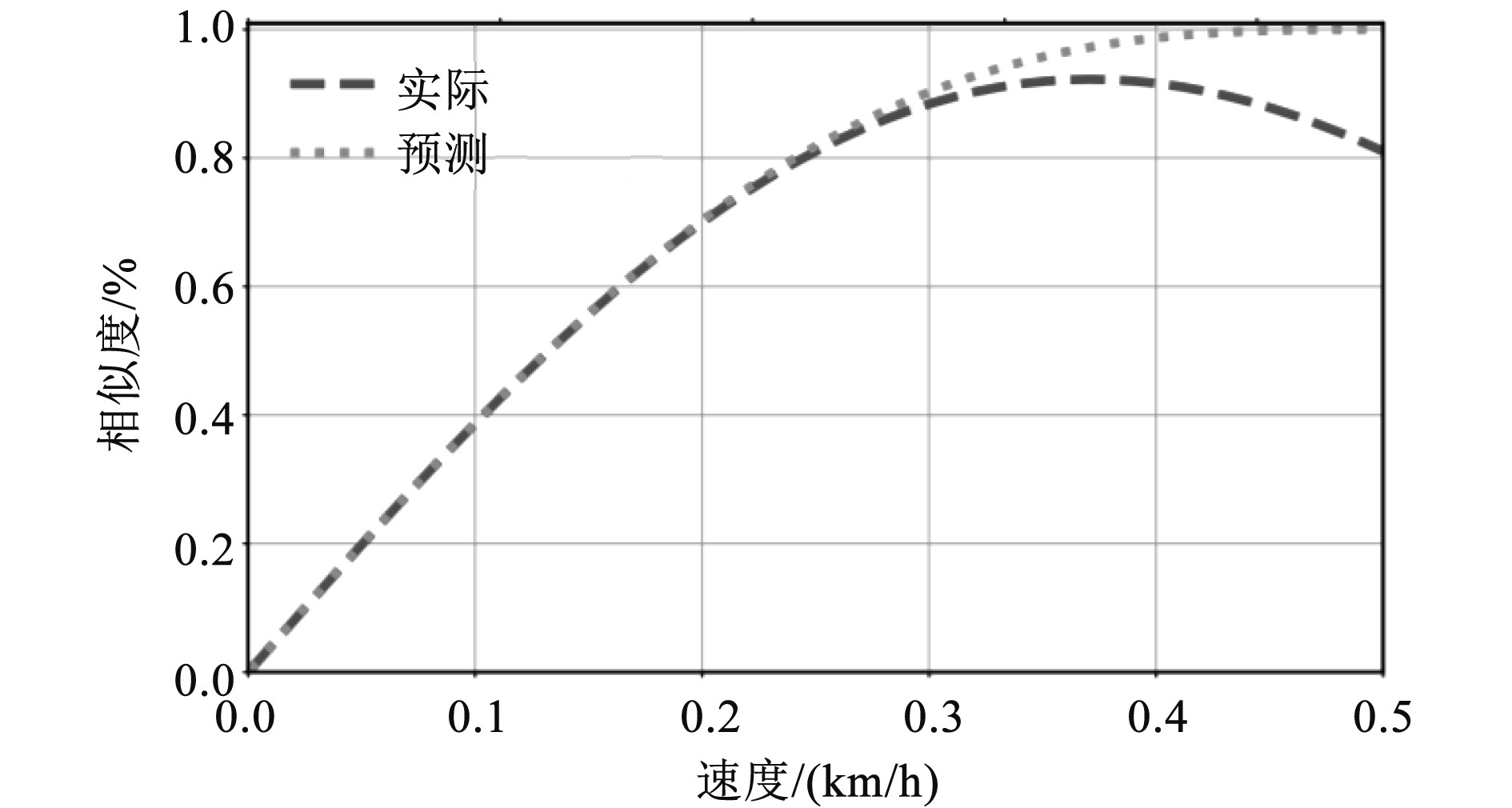图 1 实体船舶无量纲轴向速度的实际与预测相似度曲线 Fig. 1 Actual and predicted similarity curves for dimensionless axial velocities of solid ships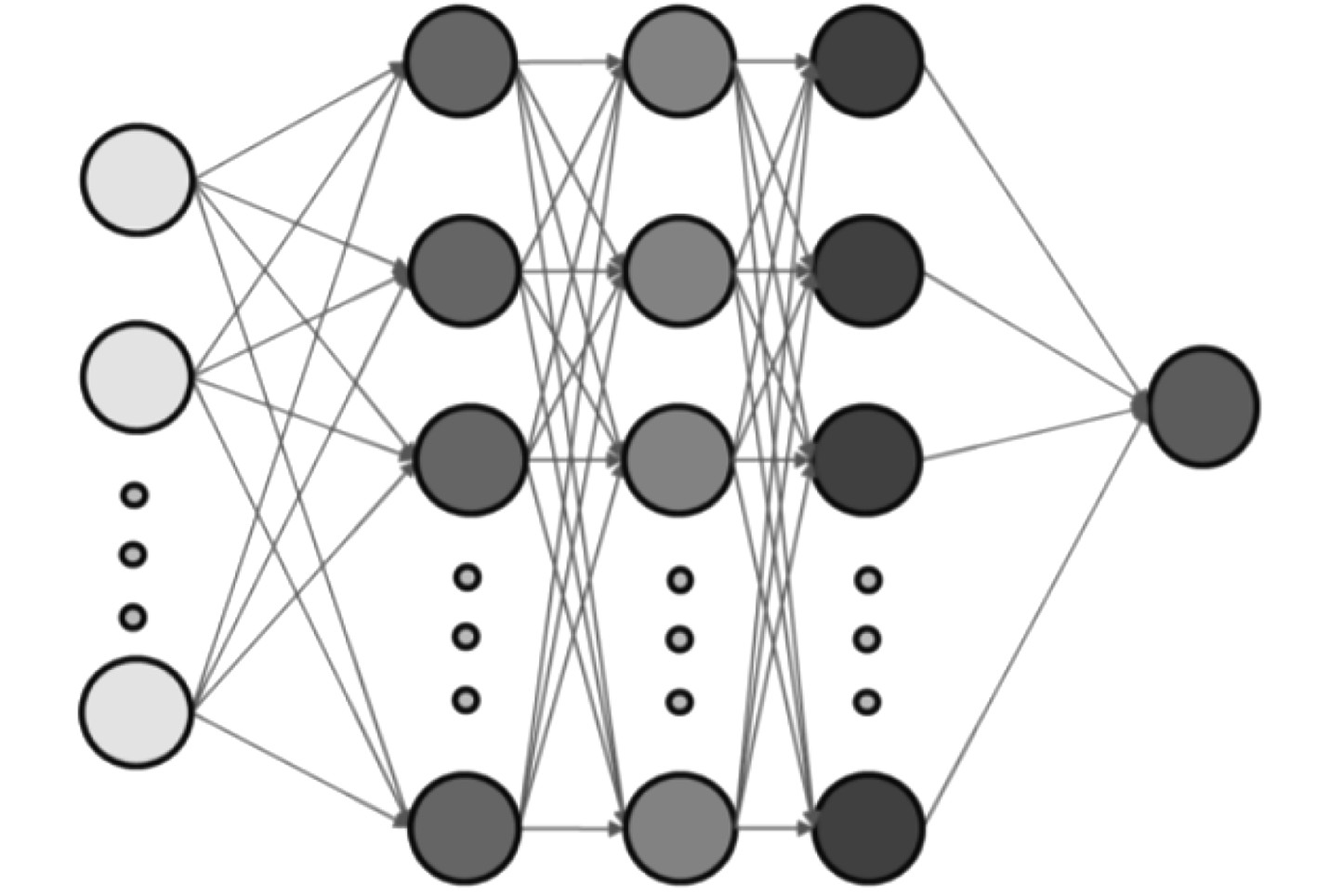图 2 船模推进系统的输入输出结构框图 Fig. 2 Block diagram of input and output structure of ship model propulsion system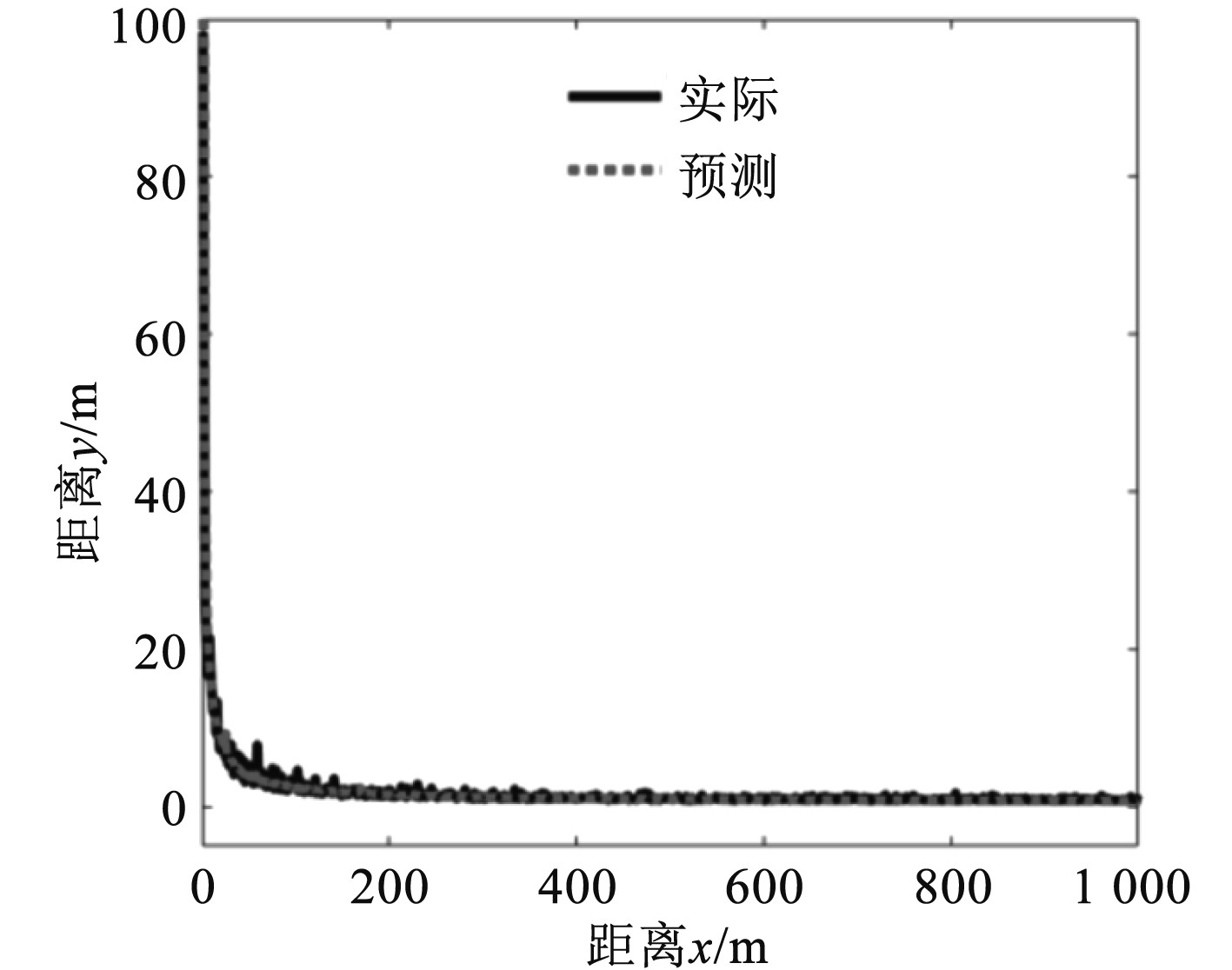图 3 实体船舶自航试验变化曲线示意图 Fig. 3 Schematic diagram of variation curve of self navigation test of solid ship

2.3 振力数值计算结果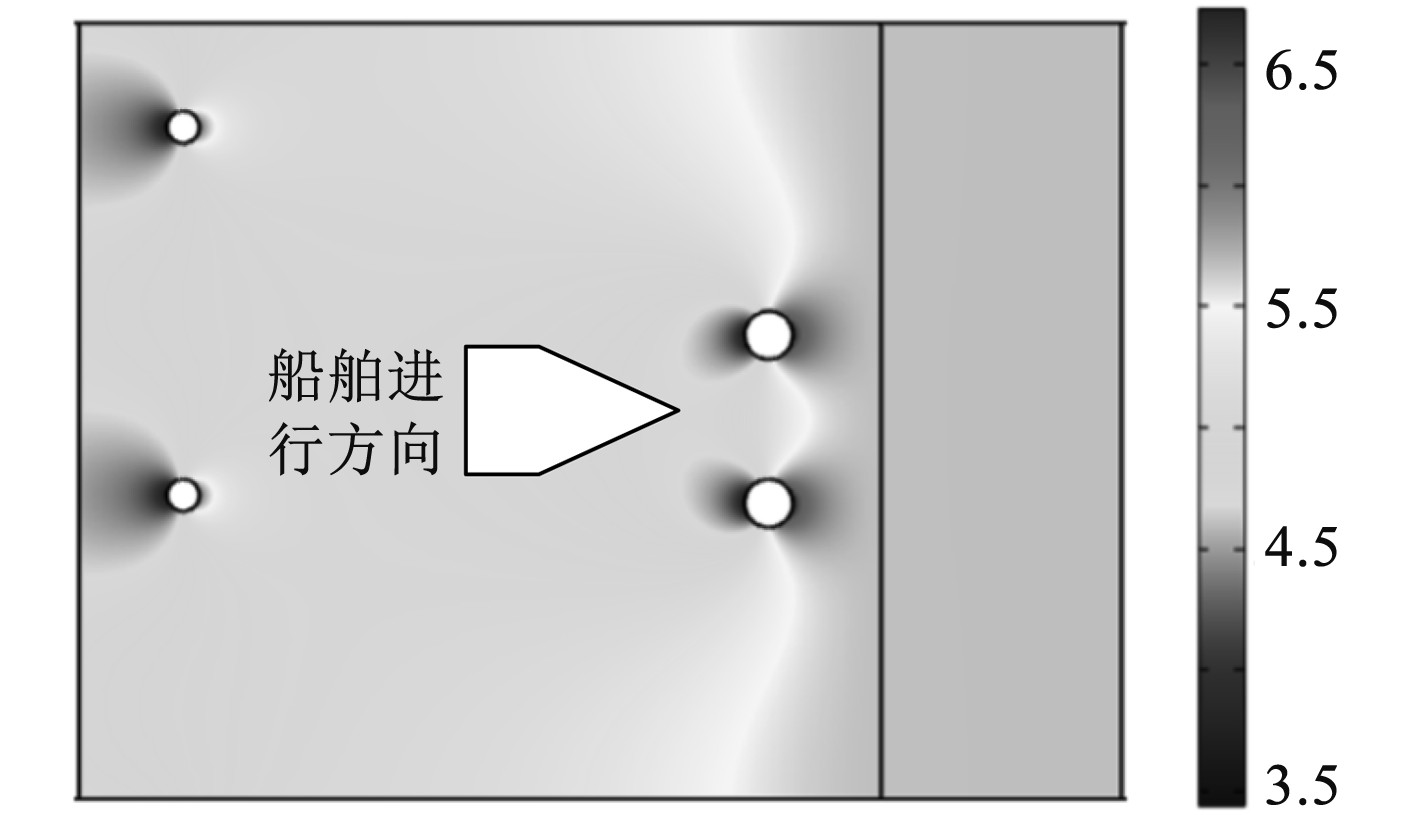图 4 实体船舶和船模桨盘面在自航条件下0.3倍直径处的无量纲轴向速度对比 Fig. 4 Comparison of dimensionless axial velocity at 0.3 times diameter between solid ship and ship model propeller disk under self navigation condition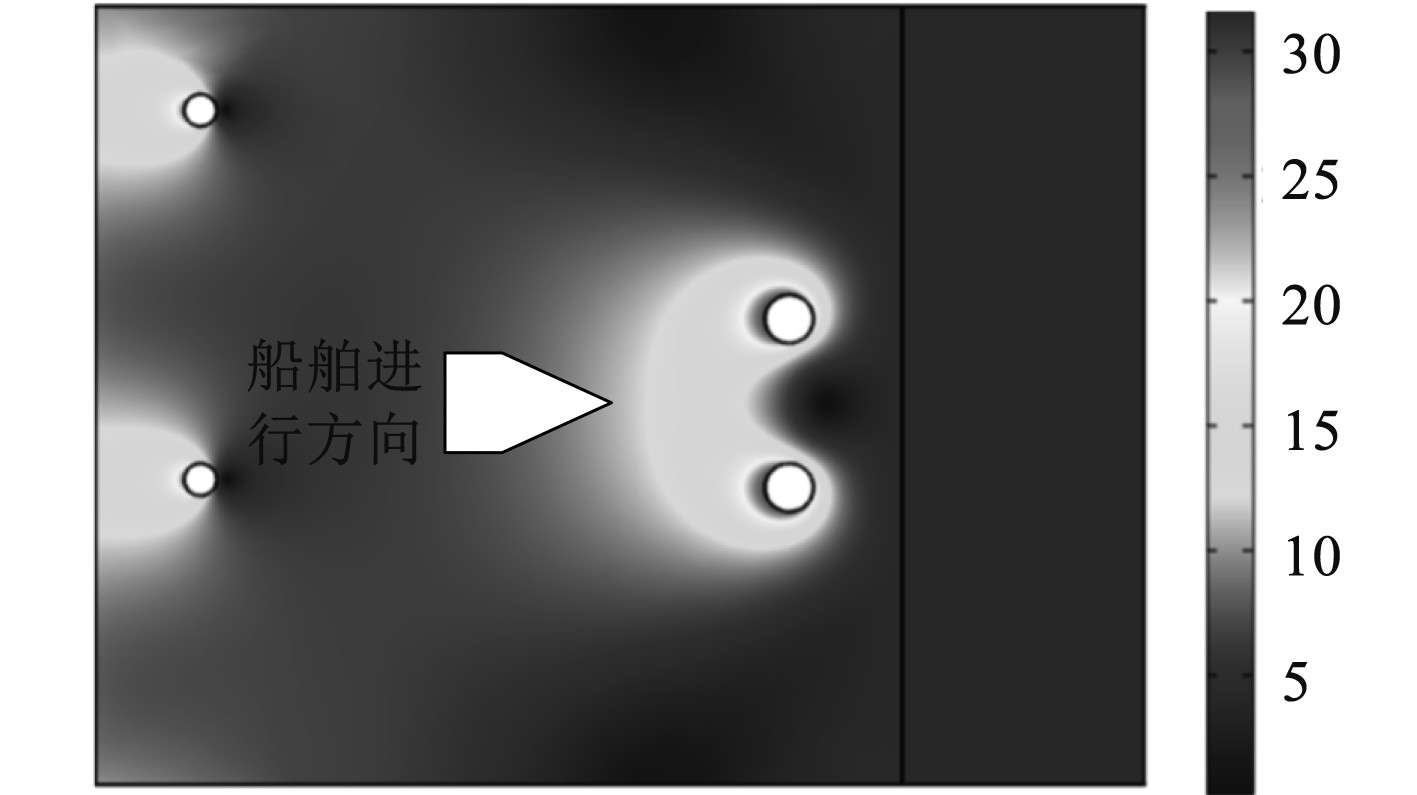图 5 自由液面波形情况下的速度变化图 Fig. 5 Velocity change diagram in the case of free surface waveform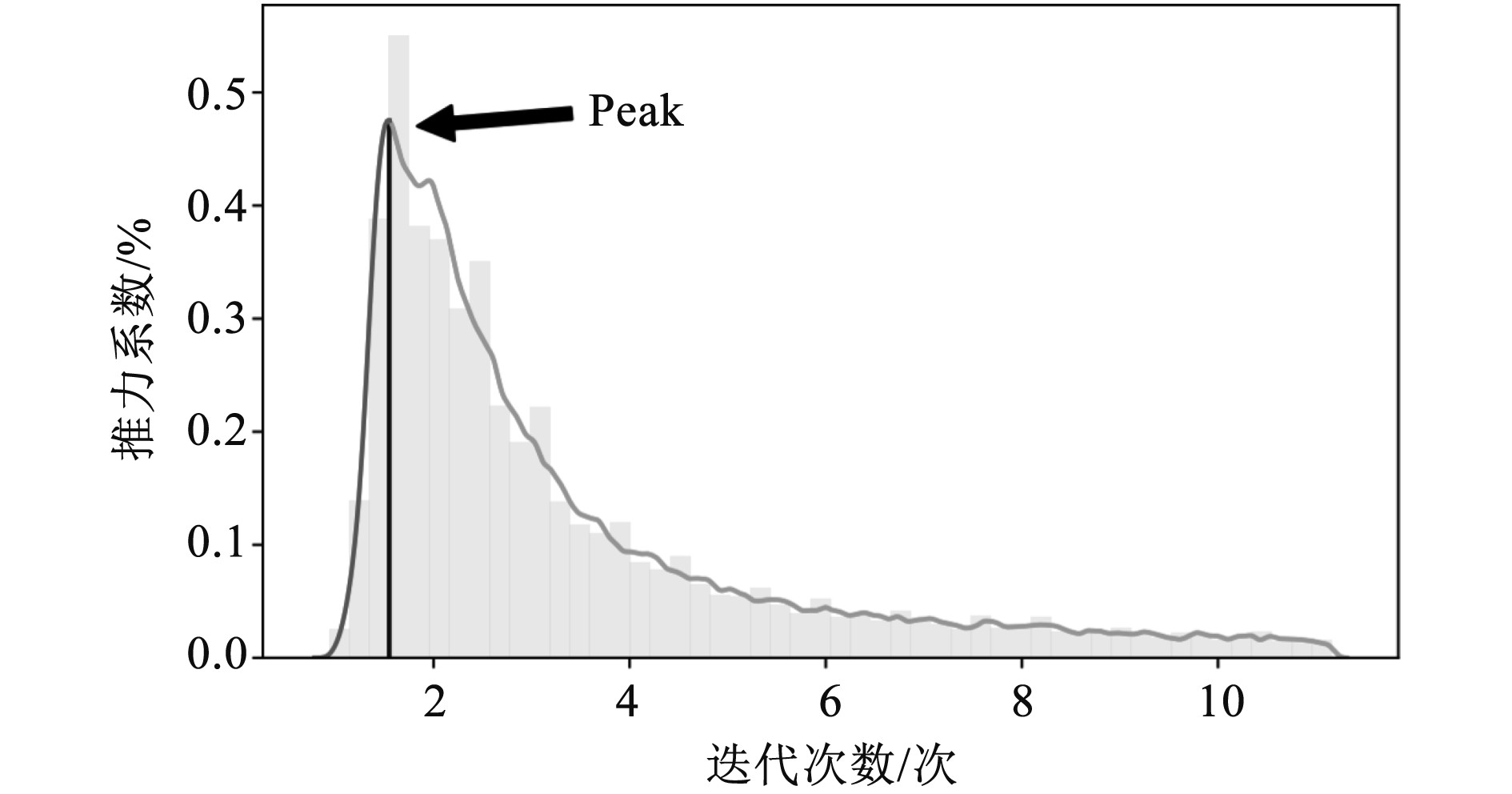图 6 单片桨叶推力系数的脉动曲线示意图 Fig. 6 Schematic diagram of pulsation curve of thrust coefficient of single blade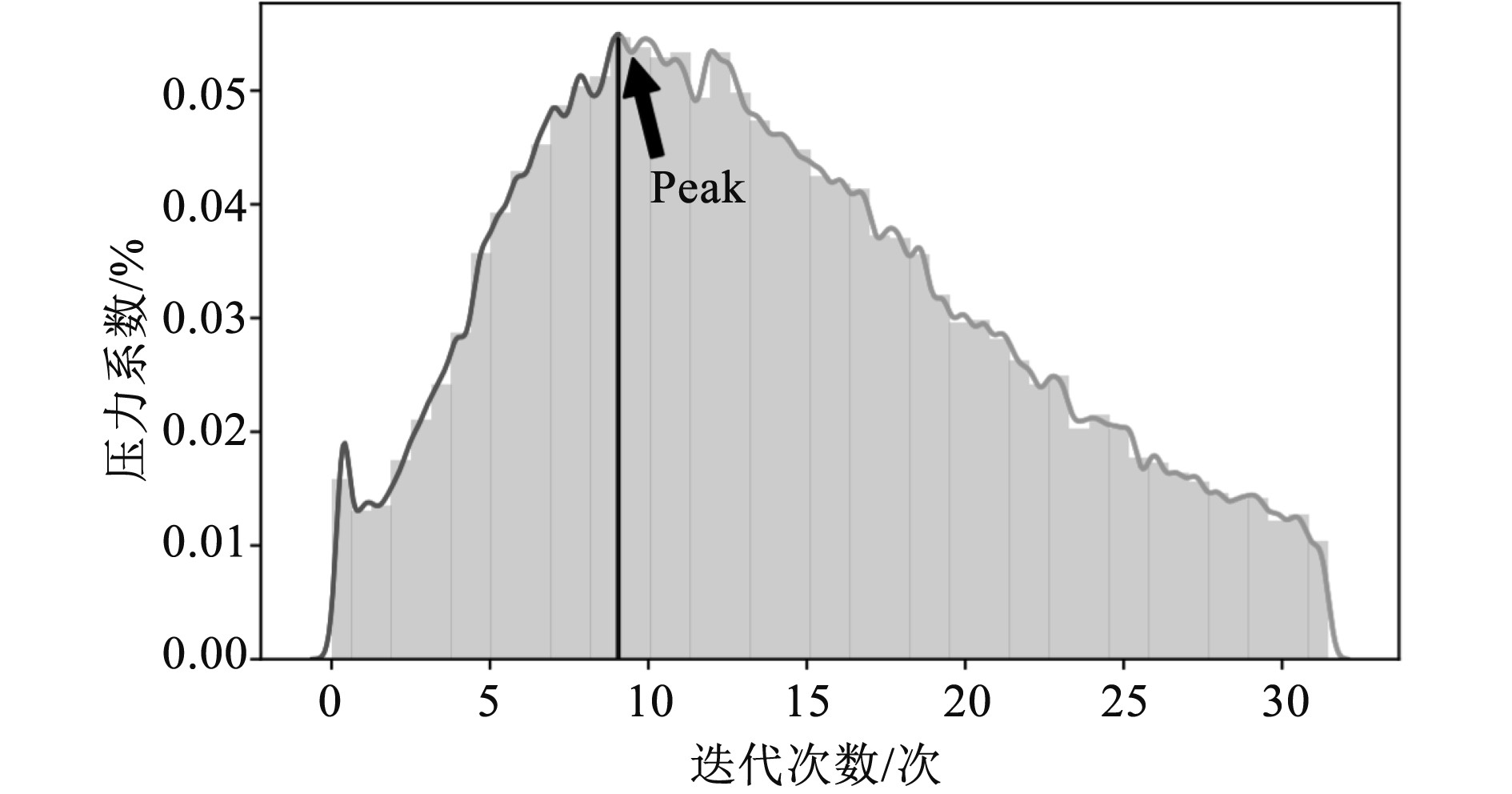图 7 实体船舶与船模各测点的脉动压力系数均值 Fig. 7 Mean value of fluctuating pressure coefficient at each measuring point of solid ship and ship model

3 结　语

  丁宁, 高占峰, 肖宇. 船舶振动响应计算中螺旋桨脉动压力的模拟方法研究[J]. 船舶, 2021(4): 37-42.  王路才, 周其斗. 桨叶振动对螺旋桨垂向激励下潜艇结构振动与声辐射的影响[J]. 中国舰船研究, 2020(5): 161-166.  李亮, 刘登成, 洪方文, 等. 风浪作用下船体纵摇运动对螺旋桨激振力特性影响分析[J]. 中国造船, 2020(2): 1-13. DOI:10.3969/j.issn.1000-4882.2020.02.001  刘小磊, 林威, 陈海明. 船艇高速螺旋桨引起的船体振动分析与解决措施[J]. 广东造船, 2019(4): 34-37. DOI:10.3969/j.issn.2095-6622.2019.04.010  张雪冰, 田佳彬, 王隽, 等. 螺旋桨非定常力对推力轴承油膜刚度影响的理论与试验研究[J]. 中国造船, 2019(2): 164-170. DOI:10.3969/j.issn.1000-4882.2019.02.016  王诗洋, 汤佳敏, 王文全, 等. 艇桨一体的螺旋桨激振力和水动力噪声数值预报[J]. 中国舰船研究, 2019(1): 43-51.  姚慧岚, 张怀新. 理论和数值方法预报的船舶姿态改变对螺旋桨非定常轴承力的影响[J]. 大连海事大学学报, 2018(3): 1-8.